﻿ Mathematical Modeling of Vertical Position Stability of QuadrocopterPublications are Open
Access in this journal
Article Versions
Export Article
• Normal Style
• MLA Style
• APA Style
• Chicago Style
Research Article
Open Access Peer-reviewed

Mathematical Modeling of Vertical Position Stability of Quadrocopter

Michal Kelemen, Ivan Virgala , Tomáš Lipták, Milan Škurka
Journal of Automation and Control. 2017, 5(2), 60-63. DOI: 10.12691/automation-5-2-6
Published online: December 11, 2017

Abstract

The paper deals with investigation of position stability of quadrocopter in vertical axis. At first, dynamic model of quadrocopter is introduced. In the paper are derived relations for control error in steady state for PD and PID controller. Based on dynamic model the PID controller is designed by optimizing method. Using designed controller the quadrocopter stability in vertical axis was simulated in software Matlab. The results of the simulation are discussed in the conclusion.

1. Introduction

The development and investigation of autonomous flight systems have increased lately because of the increasing amount of applications of unmanned aerial vehicle (UAV) in military fields such as intelligence, surveillance, and reconnaissance missions, and in civil fields like aerial surveillance, aerial photography and video, firefighting, and many others that are emerging 1, 2. The quadrocopter it’s a flying object, which flies with a help by four propellers. The quadcopter is popular concept for an drone, because of its properties. The major advantages for the quadcopter is its ability to hover, and takeoff vertically. This makes the quadcopter useful for many applications and allows it to be operated in nearly any environments.

• Figure 1. The principle of the quadrocopter flight

Based on traditional convention, Euler angle transformation are defined by ψ, θ, Φ which are yaw, pitch and roll angles, respectively. Combined transformation matrix from body coordinate to the world coordinate is obtained by three rotations, see Figure 1.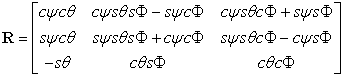(1)

where cψ represents cos(ψ) and sθ represents sinθ. The equation of motion including thrust force, hub force, drag moment, rolling moment, pitching moment, yawing moment and forces along x, y, z axis can be summarized as follows: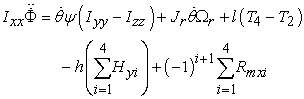(2)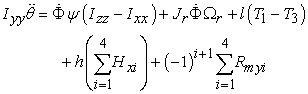(3)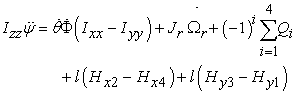(4)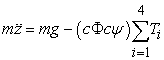(5)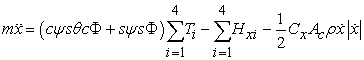(6)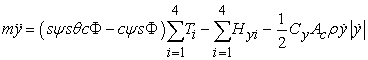(7)

where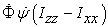is body gyro effect,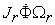is propeller gyro effect,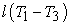is pitch actuators action,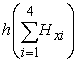hub moment due to forward flight,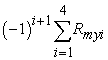is rolling moment due to sideward flight,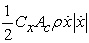is friction.

For vertical position stability of quadrocopter will be used following state space description: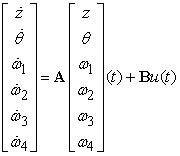(8)

By considering that motor torque is T, we can write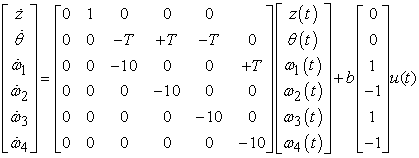(9)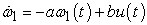(10)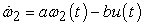(11)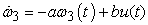(12)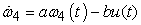(14)

By Laplace transformation we get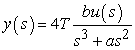(14)

In general, the system can be described as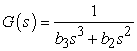(15)

The feedback control scheme of PD controller is shown in the Figure 2.

• Figure 2. Control scheme of PD controller

where D(s) is disturbance for example wind flow in vertical direction. The aim of this control is to following of desired variable zt(s). From the equation (15) is obvious, that controlled system is not asymptotically stable (level of astatism is 2).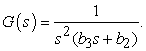(16)

We would like to control error e(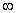) = 0.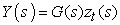(17)

The transfer function of PD controller is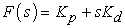(18)

By elementary mathematical operations we get following relation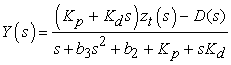(19)

The relation between control error and controlled variable is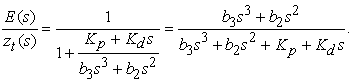(20)

The relation between control error and disturbance is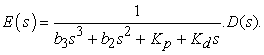(21)

Final equation explaining relation between control error, controlled variable and disturbance is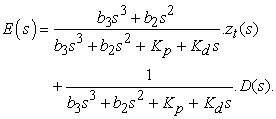(22)

Control error in steady state is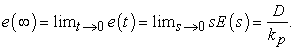(23)

From the equation (23) is obvious that PD controller causes control error differing from 0.

Now, there will be shown how would operates PID controller. The process of determining of control error in steady state is the same. Using PID controller we obtain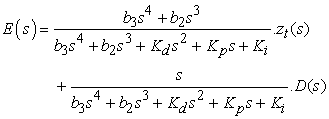(24)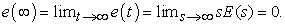(25)

The equation (25) shows that by using PID controller one can obtain zero control error in steady state.

4. Design of Controller

The function of quadrocopter controller is to control of its position as well as control of its orientation. There are a lot of attitudes to quadrocopter designing which were discussed in the past like for example PID control 1, 3, LQR control 4, H∞ control 5, 6, 7.

In our study PID controller will be designed. The inputs of the system are four independent speeds of propellers and their rotations about the axes of quadrocopter arms. There are several methods how to find optimal constants of controller. In this study will be used method which seeks extremum of the function. This method is optimizing method which works numerically with quadrocopter dynamic model. We would like to cost function C(φ) which penalizes high error as well as error over duration of the time. This cost function is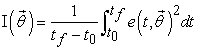(26)

e is error which would be minimal. The algorithm of method iteratively improve parameter vector by defining parameter update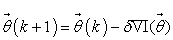(27)

where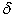is step size which dictates how much we adjust our parameter vector at each step of the iteration. Using this, we can approximate the derivative with respect to any of the gains numerically, simply by computing.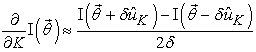(28)

where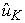is the unit vector in the K direction. Using this function we can minimizing cost function and get locally optimal PID parameters.

5. Simulations and Results

The simulation in software Matlab 2014b is done. There are used following parameters for simulation: weight = 0.5 kg; length of quadrocopter arm = 0.3 m; simulation time = 10 s; gravitational acceleration = 9.81 m/s2; moment of inertia (diagonal matrix) = 0.005, 0.005, 0,0025 kgm2. For PID controller are used following constants: Kd = 4, Kp = 3 and Ki = 5.5.

The results of the simulation in the following figures are shown.

• Figure 3. Rotation in respective directions
• Figure 4. Rotation in respective directions
• Figure 5. Motion of Center of Gravity
• Figure 6. Revolutions of Quadrocopter Motors
• Figure 7. Velocity of Center of Gravity

As can be seen from the simulation, the vertical position of quadrocopter stabilizes after a few seconds. The Figure 8 shows animation of quadrocopter motion during its vertical stabilization.

• Figure 8. Simulation of Quadrocopter Motion

6. Conclusion

This paper presents study of quadrocopter stability in vertical axis using PID controller. Using PD controller the system would have control error differing from zero in steady state. By PID controller can be achieved zero control error. In the paper was used optimizing method for finding out optimal PID controller parameters. The simulation shows, that angular velocity as well as rotation are zero in steady state.

Acknowledgements

This paper was supported by project VEGA1/0872/16 “Research of synthetic and biological inspired locomotion of mechatronic systems in rugged terrain”.

References

  S. Bouabdallah, P. Murrieri, R. Siegwart, Towards Autonomous Indoor Micro VTOL, Autonomous Robots, 2005. In article View Article  L. Nagy, Bristled Micromachines, Acta Mechanica Slovaca. Volume 19, Issue 2, Pages 6-14, ISSN 1335-2393. In article  G. M. Hoffmann, Quadrotor helicopter flight dynamics and control: Theory and experiment, In Proceedings of the AIAA Guidance, Navigation, and Control Conference, pp. 1-20, 2007. In article View Article  P. Castillo, R. Lozano, A. Dzul, Stabilization of a mini rotorcraft with four rotors, Control Systems, pp. 45-55, 2005. In article View Article  M. Chen, M. Huzmezan, A Simulation Model and H( Loop Shaping Control of a Quad Rotor Unmanned Air Vehicle, Modelling, Simulation, and Optimization, pp. 320-325, 2003. In article  M. De Lellis, Modeling, Identification and Control of a Quadrotor Aircraft, Czech Technical University in Prague, 2011. In article PubMed  PubMed  F. Duchon, J. Rodina, P. Hubinsky, D. Rau, J. Kostros, “Generalized dynamic model and control of ambiguous mono axial vehicle robot”, International Journal of Advanced Robotic Systems (2016). In article View Article

Published with license by Science and Education Publishing, Copyright © 2017 Michal Kelemen, Ivan Virgala, Tomáš Lipták and Milan ŠkurkaThis work is licensed under a Creative Commons Attribution 4.0 International License. To view a copy of this license, visit http://creativecommons.org/licenses/by/4.0/